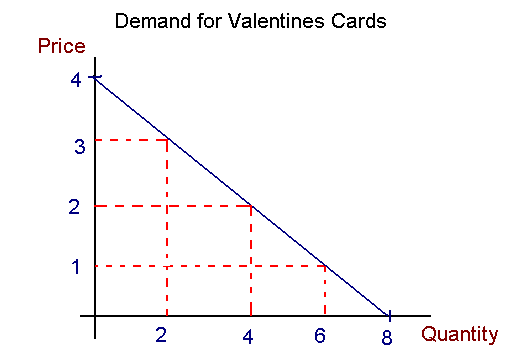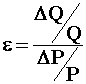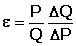Temple University

Economics 51 and 52

# Demand Elasticity

08/16/10 03:38 PM

After filling in your answers and before clicking submit, you should print out this page for your records.  You will need to bring it with you in the event that you believe you submitted the homework, but I don't record a grade for you.

The demand curve for Valentine's Day cards is shown below:The picture is drawn to scale. That is, using your ruler carefully, you can find X,Y coordinates from the picture.

Name:

1. What is total revenue when Price = 3?

2. What is total revenue when Price = 2?

3. What is total revenue when Price = 1?

4. What is the elasticity of demand if  P = 1 ?

5. What is the elasticity of demand if price is P = 2 ?

6. What is the elasticity of demand if price is P = 3?

7. What is the elasticity of demand if price is raised from P=1 to P=2?

8. What is the change in total revenue when price is
changed from  P = 1 to P = 2?
Is the change in revenue consistent with your answer to 7.? Yes  No

9. What is the elasticity of demand if price is raised from P=2 to P=3?

10. What is the change in total revenue when price is
changed from P=2 to P=3?   Is the change in revenue consistent with your answer to 9.? Yes  No

11. Do you see any apparent discrepancies in your answers? (Yes or No)

Hint #1: The price elasticity of demand isHint #2: The first hint can be rearranged to give.  On the right hand side you have the ratio of a particular P to a corresponding Q times the inverse of the slope of the demand curve.